# Computed Results

A listing of results that are computed in HyperLife.

Principal Stress and Principal Strain
For a given stress/strain tensor S, the three principals are obtained as the roots of the cubic equation below: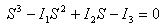Where: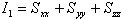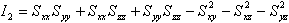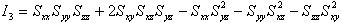In the case of a strain tensor, the shear components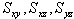are in the “Tensor shear Strain” format. Various solvers output shear strains in one of the two formats: “Engineering shear strain” and “Tensor shear strain”. “Engineering shear strain” is twice that of “Tensor shear strain”. All solver data that has shear strains in “Engineering shear strain” are converted to “Tensor shear strain” before calculating the principals. This includes some solutions from Nastran, Abaqus, and ANSYS.
vonMises Stress
=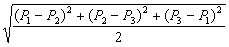Where: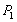,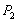,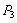represent the principal stresses as calculated above for a stress tensor.
vonMises Strain
=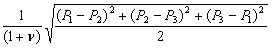Where:,,represent the principal strains as calculated above for a strain tensor.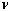is the Poisson ratio set to a default value of 0.3 by HyperLife, however for the following solver results a value a of 0.5 is used:
• Abaqus ODB
• ANSYS (Plastic and Creep Strain)
• LS-DYNA
• Nastran
• Marc t16
Signed vonMises
The sign of the signed vonMises is determined by the sign of that principal which has the biggest absolute value.
Pressure
The pressure is generated by the average of the 3 normal stresses:
Pr = -(StressX + StressY + StressZ) / 3.0.
Intensity
The absolute difference between Major and Minor stresses:
Intensity = abs (P1-P3)
Max Shear
The maximum shear stress/strain:
Max shear = abs(P1(major) - P3(minor))/2
Tresca
Computed as the Maximum of the following:
abs(P1-P2), abs(P1-P3), abs (P2-P3)
Lodeparam xi
= [(27/2) *(P1+pressure) * (P2+pressure) * (P3+pressure)] / (vonMises* vonMises * vonMises);
Lodeparam theta
= 1 – [2 * Pi * arccos(lodeparamxi)];
Triaxiality
= -pressure/VonMises
Absolute Max Principal
The largest of the three principal stresses or strains in absolute terms is contoured, preserving the sign. In other words, Absolute Max Principal is the extreme of the three principal stresses (or strains).

## References

VonMises Strain Calculation - Papers

Yu Yang, Wang Ningfei, ZhangPing, Three-dimensional Damage Analysis of Free Loading Solid Propellant Grains Subjected to Low Temperature Loading. School of Mechano-Electronics Engineering, Beijing Institute of Technology, Beijing 100081, China.